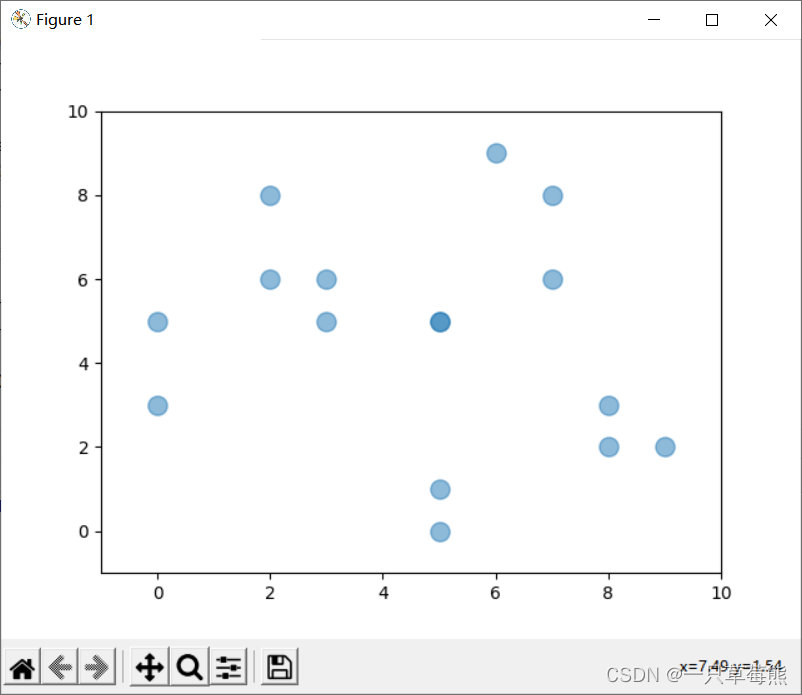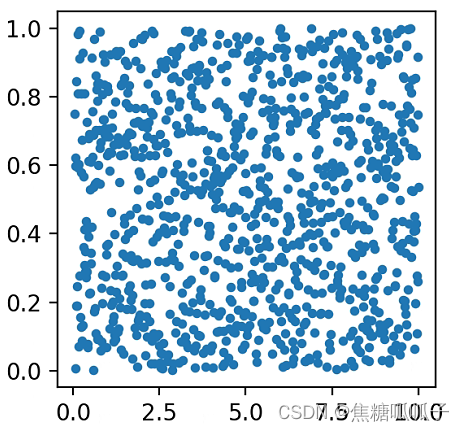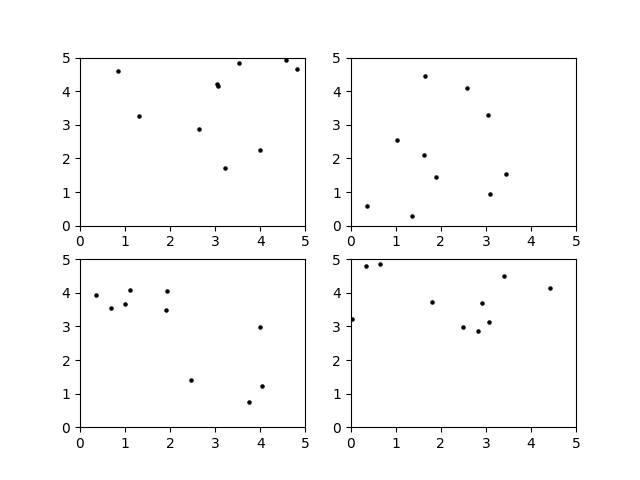• python绘制散点分布图
千次阅读
2022-05-04 16:26:51
##### Python绘制散点图，并输出散点的分布。

import matplotlib.pyplot as plt
import numpy as np

n = 16 #图中点的个数
X = np.random.randint(0, 10, n) #点的横坐标
Y = np.random.randint(0, 10, n) #点的纵坐标

plt.scatter(X, Y, s=120, c=None, alpha=0.5) #绘制散点图
#X，Y:散点的坐标
#s:表示的是散点的大小，默认大小是20。
#c:表示的是散点的颜色，默认颜色是蓝色'b'。
#alpha:散点透明度，取值[0, 1]之间的值；0表示完全透明，1则表示完全不透明。

plt.xlim(-1, 10)# 设置x轴的坐标刻度
plt.ylim(-1, 10)# 设置y轴的坐标刻度

plt.show()



输出结果：python
更多相关内容
• 主要为大家详细介绍了python绘制地震散点图的相关方法，具有一定的参考价值，感兴趣的小伙伴们可以参考一下
• python绘制散点图

## 前言

散点图是指在回归分析中，数据点在直角坐标系平面上的分布图，散点图表示因变量随自变量变化的大致趋势，据此可以选择合适的函数对数据点进行拟合

用两组数据构成多个坐标点，考察坐标点的分布，判断两变量之间是否存在某种关联或总结坐标点的分布模式。散点图将序列显示为一组点。值由点在图表中的位置表示。类别由图表中的不同标记表示。散点图通常用于比较跨类别的聚合数据。

如果需要绘制折线图，请参考我的这篇文章：

使用python绘制折线图_焦糖呱呱子的博客-CSDN博客

## 一、基本命令

plt.scatter(x,y,s,color='',cmap='',marker='',alpha='')

（x, y）是其在坐标中的位置，s表示打点的大小，color表示打点的颜色，cmap表示颜色映射表，marker表示打点的标记，alpha修改透明度。举个例子：

import matplotlib.pyplot as plt
import numpy as np
x=np.linspace(0.05,10,1000)
y=np.random.rand(1000)
fig=plt.figure(figsize=(3,3),dpi=200)
ax.scatter(x,y,s=10,)
plt.show()

运行结果：## 二、细节问题

（1）中文不显示以及负号显示异常

在前加一句：

plt.rcParams['font.sans-serif']=['...']  # ...替换成字体，常用的有SimHei（黑体），LiSu（隶书），STXingkai（行楷）等等
plt.rcParams['axes.unicode_minus'] = False   # 使坐标轴刻度表签正常显示正负号

（2）多个子图共用一个colorbar的排版问题

大家肯定想要的是colorbar显示在子图的最下方。

此时应当将colorbar单独看成一个子图进行绘制，使用

ax = fig.add_axes[i, j, m, n]

i, j代表本子图在figure中位置，表示距离figure左边和下边的距离。m, n为本子图的形状大小。具体参数需要读者在绘制过程中微调。

展开全文python 机器学习
• Python 有着强大的绘图库 matplotlib, 该库集成了大量的绘制函数，可以满足...利用 Python 绘制散点图与在 matlab 中操作类似，以数据集 fourclass 为例(数据集的获取方式见文章https://blog.csdn.net/weixin_432525

🏳️‍🌈Python 有着强大的绘图库 matplotlib, 该库集成了大量的绘图函数，可以满足我们平时绝大多数的绘图需求。其中，matplotlib 库是 Python 进行可视化功能的主要软件包，matplot 本是 matlab 中的绘图库，matplotlib 其实就是在 Python 实现 matplot 的功能。

🏳️‍🌈利用 Python 绘制散点图与在 matlab 中操作类似，以数据集 fourclass 为例(数据集的获取方式见文章 Python 对数据集进行归一化处理并存储为 mat 格式的文件

🏳️‍🌈样本的两个属性值作为在平面图中的二维坐标，根据样本的标签值给散点相应的颜色，代码如下：

from scipy.io import loadmat  #导入 loadmat, 用于对 mat 格式文件进行操作
import numpy as np
import matplotlib.pyplot as plt  #导入绘图操作用到的库

#读取数据得到一个字典类型数据，需要根据键名 ’data‘ 取出对应的值。
fig = plt.figure()  # 创建画布
idx_1 = np.where(data[:, -1] == 1)  # 找出标签为1的样本
idx_2 = np.where(data[:, -1] == -1)  # 找出标签为-1的样本
#绘制散点图
p1 = ax.scatter(data[idx_1, 0], data[idx_1, 1], marker='.', color='black', s=8)
p2 = ax.scatter(data[idx_2, 0], data[idx_2, 1], marker='.', color='red', s=8)
plt.show()   #显示散点图


🏳️‍🌈首先，创建一个画布，然后再调用add_subplot() 函数，111 表示这个画布中，第一行第一列中的第一个子图，add_subplot(111) 就是在创建的画布中创建第一个子图，这个子图在第一行第一列，一般只画一个图就使用 111 就可以了。

🏳️‍🌈其次，根据样本的标签找出对应的样本。fourclass 数据集是二分类数据集，如果你使用的是其他数据集，有 N 个分类，可以依此类推设置 idx_N = np.where(data[:, -1] == N) # 找出第N类

🏳️‍🌈然后，绘制散点图。以 p1 所在行的代码为例，ax.scatter() 函数第一个参数 data[idx_1, 0] 是样本的横坐标，第二个参数 data[idx_1, 1] 是样本的纵坐标，marker 表示散点的形状， color 表示颜色参数，s 表示散点的像素大小。值得注意的是，这里所绘制的散点图是二维的，碰巧我们使用的数据集也是只有两个条件属性，可以比较好的刻画样本的分布，如果你使用的数据集的条件属性大于2个，自己综合考虑选择两个代表性的属性分别作为横、纵坐标。

🏳️‍🌈最后，将创建的散点图显示在画布中，绘制的散点图如下所示：展开全文python matlab matplotlib
• # 定义画散点图的函数 def draw_scatter(n, s): """ :param n: 点的数量，整数 :param s:点的大小，整数 :return: None """ # 加载数据 data = np.loadtxt('results1.txt', encoding='utf-8', delimiter=',')...

从文件results1.txt中读取数据，results1.txt放在py文件同目录下，数据形式为八列

results1.txt数据：

1.318,3.272,0.361,0.572,0.677,3.539,3.405,4.505
3.051,4.163,1.648,4.442,0.991,3.676,2.917,3.686
3.211,1.729,1.632,2.093,4.039,1.228,0.648,4.866
4.564,4.920,3.045,3.296,1.936,4.042,0.042,3.233
4.823,4.675,1.364,0.282,1.103,4.087,1.815,3.731
2.640,2.864,3.450,1.541,3.739,0.743,4.417,4.136
3.035,4.213,3.089,0.951,2.467,1.409,3.079,3.131
3.993,2.260,1.028,2.534,1.897,3.477,0.346,4.802
3.520,4.848,1.891,1.436,3.998,2.978,2.825,2.861
0.844,4.590,2.579,4.081,0.349,3.923,2.503,2.968

代码：

import matplotlib.pyplot as plt
import numpy as np

# 定义画散点图的函数
def draw_scatter(n, s):
"""
:param n: 点的数量，整数
:param s:点的大小，整数
:return: None
"""
# 加载数据
data = np.loadtxt('results1.txt', encoding='utf-8', delimiter=',')
# 通过切片获取横坐标x1，data[:, 0]表示第一列数据
x1 = data[:, 0]
# 通过切片获取纵坐标R，data[:, 1]表示第二列数据
y1 = data[:, 1]
x2 = data[:, 2]
y2 = data[:, 3]
x3 = data[:, 4]
y3 = data[:, 5]
x4 = data[:, 6]
y4 = data[:, 7]

# 创建画图窗口
fig = plt.figure()
# 设置标题
#ax.set_title('Scatter diagram')
# 设置横坐标名称
#ax.set_xlabel('gamma-value')
# 设置纵坐标名称
#ax.set_ylabel('R-value')
# 将画图窗口分成2行2列，选择第1块区域作子图
ax1 = fig.add_subplot(2, 2, 1)
# 画散点图
ax1.scatter(x1, y1, s=s, c='k', marker='.')
# 调整横坐标的上下界
plt.xlim(xmax=5, xmin=0)
plt.ylim(ymax=5, ymin=0)
# 将画图窗口分成2行2列，选择第2块区域作子图
ax2 = fig.add_subplot(2, 2, 2)
# 画散点图
ax2.scatter(x2, y2, s=s, c='k', marker='.')
# 调整横坐标的上下界
plt.xlim(xmax=5, xmin=0)
plt.ylim(ymax=5, ymin=0)
# 将画图窗口分成2行2列，选择第3块区域作子图
ax3 = fig.add_subplot(2, 2, 3)
# 画散点图
ax3.scatter(x3, y3, s=s, c='k', marker='.')
# 调整横坐标的上下界
plt.xlim(xmax=5, xmin=0)
plt.ylim(ymax=5, ymin=0)
# 将画图窗口分成2行2列，选择第4块区域作子图
ax4 = fig.add_subplot(2, 2, 4)
# 画散点图
ax4.scatter(x4, y4, s=s, c='k', marker='.')
# 调整横坐标的上下界
plt.xlim(xmax=5, xmin=0)
plt.ylim(ymax=5, ymin=0)
# 显示
plt.show()

# 主模块
if __name__ == "__main__":
# 运行
draw_scatter(n=10, s=20)

输出结果：展开全文python
• """使用scatter()绘制散点图""" import matplotlib.pyplot as plt plt.scatter(2, 4) plt.show() 下面来设置输出的样式：添加标题，给轴加上标签，并确保所有文本都大到能够看清。并使用scatter()绘制一系列点 ""...
• ## Python matplotlib绘制散点图

万次阅读 多人点赞 2020-11-03 23:50:08
• 主要为大家详细介绍了Python Matplotlib实现三维数据的散点图绘制，具有一定的参考价值，感兴趣的小伙伴们可以参考一下
• 最初遇到这个问题的时候，找到的大答案是绘制contour，比如： http://stackoverflow.com/questions/24119920/how-to-plot-a-density-map-in-python?rq=1​ 这当然很漂亮，但是这需要有三列数字来标示一个平面；...python
• 示例：散点图最常见的散点图之一是x-y散点图。下面的代码会大致告诉你一个matplotlib是如何工作的，你会看到如何一点点建立起一个散点图。我们正在使用点的x和y位置的一些构成数据。运行下面的代码，然后我们将解释...
• python绘制散点图并标记序号的方法实现二维平面上散点的绘制，并可以给每个散点标记序号或者名称：import numpy as npimport matplotlib.pyplot as pltx=[2.3,4.5,3,7,6.5,4,5.3]y=[5,4,7,5,5.3,5.5,6.2]n=np.arange...
• 大家平时为了直观地显示数据的分布情况，在画散点图的时候，简单地把数据点用圆点标出来，像这样： 这样： 还有这样： 然而今天我想给大家展示的散点图，或许没有那么直观地反映数据的分布情况，不够实用，但是真的...
• 如何用python绘制散点图呢？思路其实不复杂： 1.绘制正样本，使用“+”图标 2.在同一张上绘制负样本，使用“o”图标 3.展示正负样本 import numpy as np import matplotlib.pyplot as plt # # 载入数据 # data = ...二分类
• 他可以绘制各种图形，可是最近最的一个小程序，得到一些三维的数据点图，就学习了下python中的matplotlib模块，如何绘制三维图形。初学者，可能对这些第三方库安装有一定的小问题，对于一些安装第三方库经验较少的...
• 超详细的Python matplotlib 绘制柱状_编程简单学的博客- 这么详细的Python matplotlib 绘制图形 还不赶紧收藏_编程简单学的博客 这么详细的Python matplotlib底层原理浅析_编程简单学的博客- python入门到进阶...python
• 散点图是指在数理统计回归分析中，数据点在直角坐标系平面上的分布图散点图表示因变量随自变量而变化的大致趋势，由此趋势可以选择合适的函数进行经验分布的拟合，进而找到变量之间的函数关系。 散点图有什么用处...pyecharts Python数据分析
• 显示了数据中各组之间最佳拟合线的差异。 参考代码： import seaborn as sns sns.set(style="darkgrid") tips = sns.load_dataset("tips") g = sns.jointplot("total_bill", "tip", data=tips,kind="reg", ...python matplotlib colormap 线性回归
• `python import numpy as np import matplotlib.pyplot as plt import os #导入os库 x=np.linspace(0, 10, 30)#产生0-10之间30个元素的等差数列 noise=np.random.randn(30)#产生30个标准正态分布的元素 y1=x**2+2*...python 机器学习
• import matplotlib.pyplot as plt from mpl_toolkits.mplot3d import Axes3D import numpy as np ... 使数据分布均匀(vmin, vmax). ''' return (vmax - vmin)*np.random.rand(n) + vmin fig = plt.figu.python
• 前言上篇文章介绍了使用matplotlib绘制折线，参考：...一、matplotlib绘制散点图# coding=utf-8import matplotlib.pyplot as pltyears = [2009, 2010, 2011, 2012, 2013, 201...
• y=np.random.standard_normal((600,2))表示随机生成一个标准正态分布形状是600*2的数组，如所示2绘制散点图（使用plot）。plt.figure(figsize=(8,5))表示绘制图形的画板尺寸为8*5；plt.plot(y[:,0],y[:,1],'ro')...
• 散点图是指在数理统计回归分析中，数据点在直角坐标系平面上的分布图散点图表示因变量随自变量而变化的大致趋势，由此趋势可以选择合适的函数进行经验分布的拟合，进而找到变量之间的函数关系。 二、散点图有什么...python
• 折线plt.figure(figsize=(40, 40)) # 确定图像画布的大小plt.subplot(211) # 将画布分为两行一列plt.xlabel('Number of sample', fontsize=40) # x轴的labelplt.ylabel('Char...
• 本文实例为大家分享了python使用matplotlib画柱状散点图的具体代码，供大家参考，具体内容如下 柱状（plt.bar） 代码与注释 import numpy as np from matplotlib import pyplot as plt plt.figure(figsize=(9...
• Python matplotlib绘制散点图之基本配置--鸢尾花案例python
• Python 进行数据挖掘和分析时，只需像普通软件一样安装好 Anaconda，就可以把 Python 的环境变量、解释 器、开发环境等安装在计算机中。除此之外， Anaconda 还提供了众多科学计算的 包，如 Numpy、scpy、Pandas...python 数据挖掘
•python
• import matplotlib.pyplot as plt # 解决不能显示中文 plt.rcParams['font.sans-serif'] = ['SimHei'] plt.rcParams['axes.unicode_...plt.title("二维散点图", fontsize=24) plt.xlim(xmax=9,xmin=0) plt.ylim(ym...python...